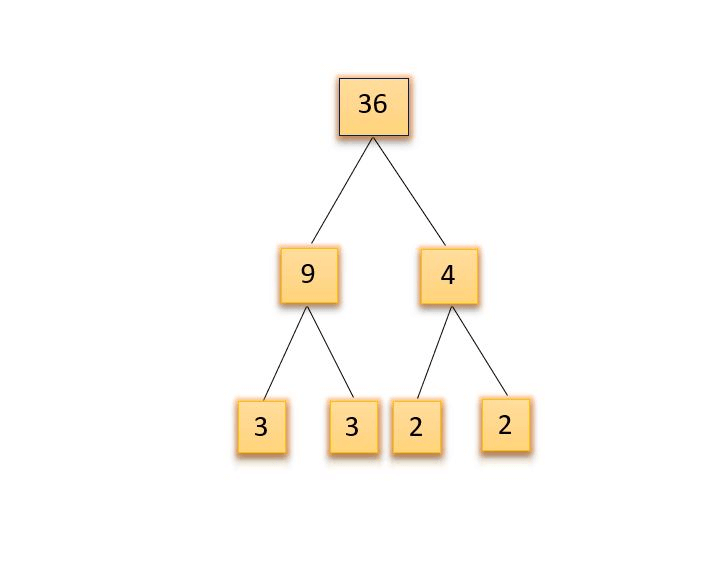# Prime Factorization and Factorization

## Find the Prime factor, Factor and Factor Pairs for a given number

HomeMath

Prime Factor

You can input a number and find the prime factor, factor and factor pair.

## What is Prime Factorization?## How to Factorize a number?• Step 1:

As we discussed about integers, we clearly notice that all integers can be written as Multiplication of two numbers. Even we can write prime number in this way.(1 x prime.no).

• Step 2:

Lets take a number xy.Try to write in multiplication of two numbers. (i.e) x * y.

• Step 3:

Next is to check whether the number x and y can be written as multiplication of two numbers.We need to repeat this step until the number reaches to prime number.

## Factor Tree• ### Factor tree of 36 is 2x2 x 3x3

First we split 36 into 9 x 4. And in each branch we have composite so our aim is to split all branch element to multiples of prime numbers.So split 9 as 3 x 3 and 4 as 2 x 2 respectively in the branch. Now at the end node all the numbers are prime numbers.So the factorization of 36 is 2 x 2 3 x 3.## Factorization table## Frequently Asked Questions on Prime Factorization